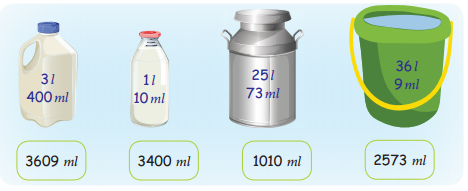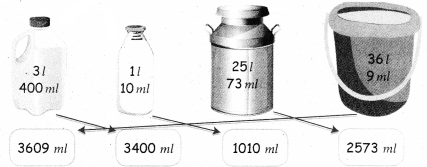Tamilnadu State Board New Syllabus Samacheer Kalvi 4th Maths Guide Pdf Term 3 Chapter 3 Measurements Ex 3.2 Textbook Questions and Answers, Notes.

## Tamilnadu Samacheer Kalvi 4th Maths Solutions Term 3 Chapter 3 Measurements Ex 3.2

Question 1.
Complete the following. First one is done for you

(i) 5l + 376 m1 = ______ ml + ______ = ______ ml
5l + 376 m1 = 5000 ml + 376 ml = 5376 ml

(ii) 3l + 735 m1 = ______ ml + ______ = ______ ml
3l + 735 m1 = 3000 ml + 735 ml = 3735 ml(iii) 4l + 43 m1 = ______ ml + ______ = ______ ml
4l + 43 m1 = 4000 ml + 43 ml = 4043 mml

(iv) 8l + 6 m1 = ______ ml + ______ = ______ ml